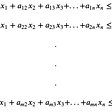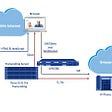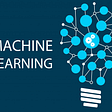# 3 Ways to build a Keras Model

The scope of this story is to build a Keras model using different ways: the sequential model, the functional model, and a custom model via sub-classing.

The sequential model:

First, building a model by passing a list of layers to it.

`model = tf.keras.Sequential([    tf.keras.layers.Flatten(input_shape=(28, 28)),    tf.keras.layers.Dense(128, activation='relu'),    tf.keras.layers.Dense(10)])`

`model = tf.keras.Sequential()model.add(tf.keras.layers.Flatten(input_shape=(28, 28)))model.add(tf.keras.layers.Dense(128, activation='relu'))model.add(tf.keras.layers.Dense(10))`

This approach only works when you have a single input and a single output.

The functional API:

Second, this approach adds arguments using parentheses; for example, the function f(x, y) becomes f(x)(y). This style is called currying in functional programming.

`input_ = tf.keras.layers.Input(shape=(28, 28))flat = tf.keras.layers.Flatten()(input_)hidden = tf.keras.layers.Dense(128, activation='relu')(flat)output_ = tf.keras.layers.Dense(10)(hidden)model = tf.keras.Model(inputs=[input_], outputs=[output_])`

This approach allows for creating complex models with: multiple inputs, auxiliary outputs, and non-sequential paths.

A custom model via sub-classing:

Finally, this approach is for research use. It allows researchers to create complex models such as: adding custom layers, skipping connections, etc.

`class CustomModel(tf.keras.Model):    def __init__(self, units=128, activation='relu', **kwargs):        super().__init__(**kwargs)        self.flat = tf.keras.layers.Flatten()        self.hidden = tf.keras.layers.Dense(units,                                            activation=activation)        self.output_ = tf.keras.layers.Dense(10)def call(self, inputs):        flat = self.flat(inputs)        hidden = self.hidden(flat)        return self.output_(hidden)model = CustomModel()`

Albeit, when it comes to serialization, this approach requires additional work.

--

--

--

## More from Mansoor Aldosari

https://github.com/booletic

Love podcasts or audiobooks? Learn on the go with our new app.

## TryHackMe Gotta Catch’em All Write-ups(Beginner Friendly and Detailed)## OverTheWire Advent writeup — Boxy## Linux Loops:## AZURE KUBERNETES SERVICE (AKS) : Industry Use Case## Simplex Method for Linear Programming## Going Native with WebRTC## What are the types of web applications?## Mansoor Aldosari

https://github.com/booletic

## Introduction to Machine Learning## Implementing PCA with NumPy## How Unsupervised Machine Learning could be used for our benefit?## Why are Python and Machine Learning Soulmates?